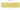# \$

## Belize Dollar

Belize uses the Belize dollar as its national currency. In order to distinguish it from other currencies denominated in dollars, it is typically abbreviated with the dollar symbol \$ or, alternatively, BZ\$. There are 100 pennies in it. The official exchange rate is 2 BZ\$ to 1 US\$.

#### how has the value of the currency changed in the last year?## USD 1 = BZD 2.011

How much is10 US dollars worth inBelize dollars?
At the current exchange rate, 10 US dollars is worth 20.11 Belize dollars
How much is50 US dollars worth inBelize dollars?
At the current exchange rate, 50 US dollars is worth 100.55 Belize dollars
How much is100 US dollars worth inBelize dollars?
At the current exchange rate, 100 US dollars is worth 201.1 Belize dollars
How much is500 US dollars worth inBelize dollars?
At the current exchange rate, 500 US dollars is worth 1,005.48 Belize dollars
How much is2,000 US dollars worth inBelize dollars?
At the current exchange rate, 2,000 US dollars is worth 4,021.9 Belize dollars
How much is10 US dollars worth inBelize dollars?
At the current exchange rate, 10 US dollars is worth 20.11 Belize dollars
How much is50 US dollars worth inBelize dollars?
At the current exchange rate, 50 US dollars is worth 100.55 Belize dollars
How much is100 US dollars worth inBelize dollars?
At the current exchange rate, 100 US dollars is worth 201.1 Belize dollars
How much is500 US dollars worth inBelize dollars?
At the current exchange rate, 500 US dollars is worth 1,005.48 Belize dollars
How much is2,000 US dollars worth inBelize dollars?
At the current exchange rate, 2,000 US dollars is worth 4,021.9 Belize dollars